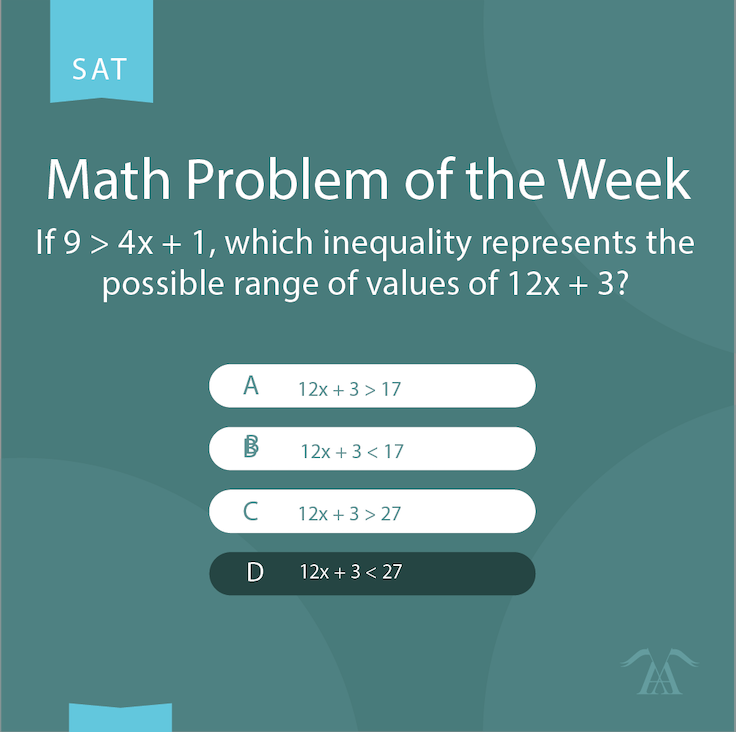## Math Answer of the WeekLet’s look at the solution to last week’s SAT Math problem:

If 9 > 4x + 1, which inequality represents the possible range of values of 12x + 3?

1. 12x + 3 > 17
2. 12x + 3 < 17
3. 12x + 3 > 27
4. 12x + 3 < 27

The correct answer is D.

While you can solve for x, then plug in the value to determine the expression for 12x + 3 (as shown below), there is a quicker solution! Multiplying 3 on both sides of the given equation is 3*(4x + 1) < 3*(9) = 12x + 3 < 27, which is D.

Note: C was the trick answer here–whichever order the inequality is written, 27 will still be the larger value.

## More from our Blog:Grammar Questions

## Grammar Question of the Week

Grammar Question of the Week: This week, let’s try a practice grammar question from the SAT. Try this question on your own, and check back to see the correct answer on Wednesday, April 20! We'll also share a complete explanation of how to answer this question on our blog.Math Questions

## Math Answer of the Week

Check out the answer to this week's Math Question of the Week, taken from the SAT. The correct answer to this question is D. In our latest blog post, we share a step-by-step guide to finding the answer to this question! Come back next Friday for another math question!